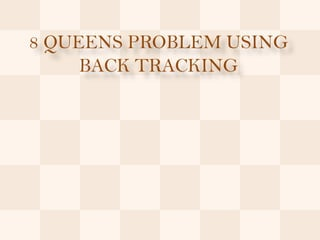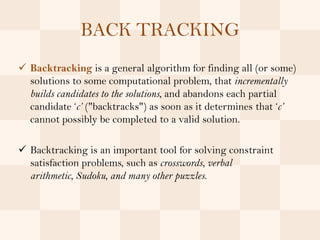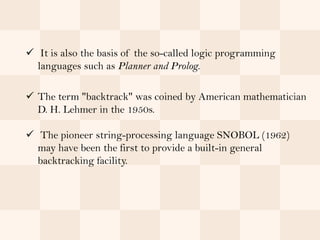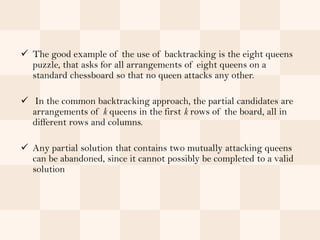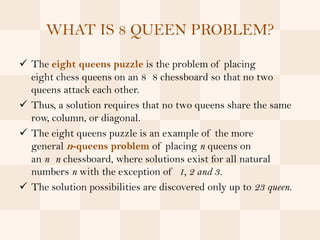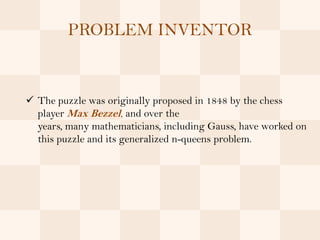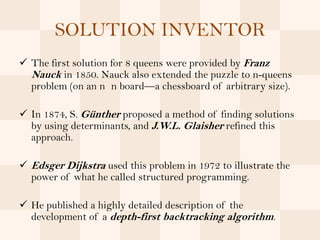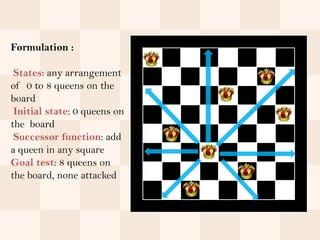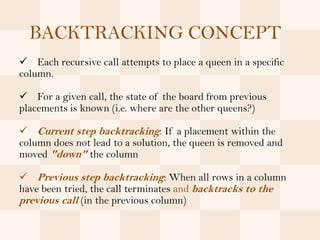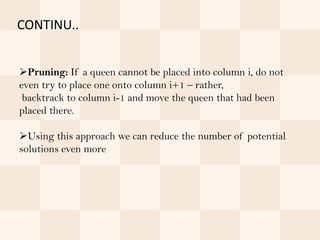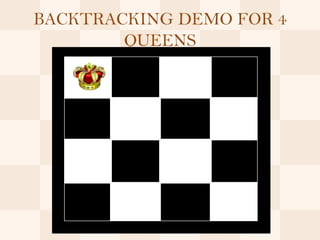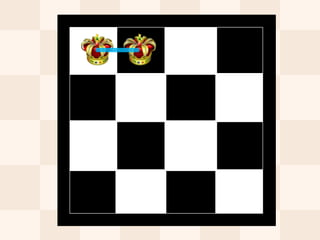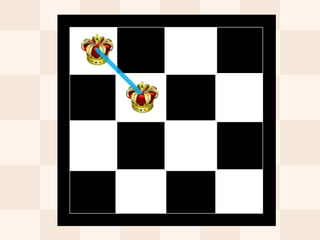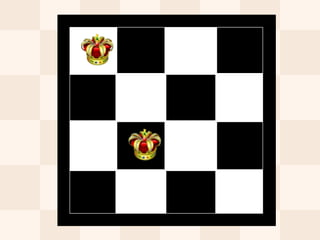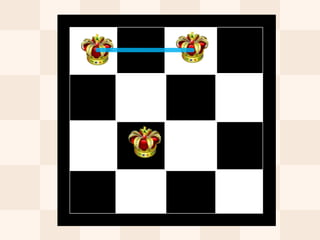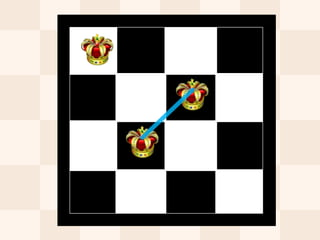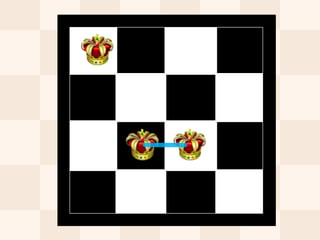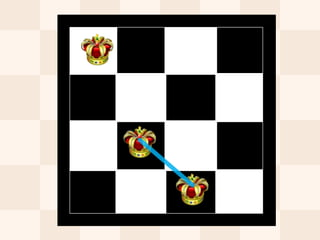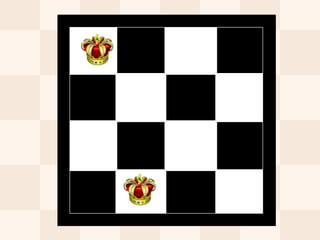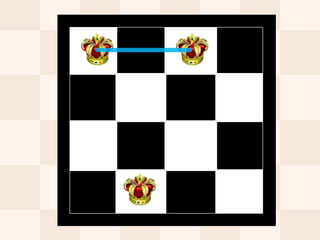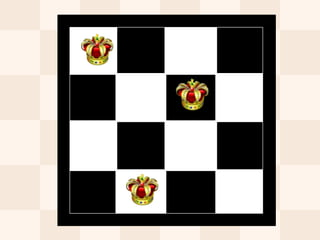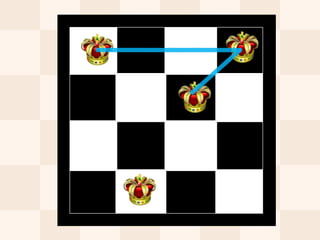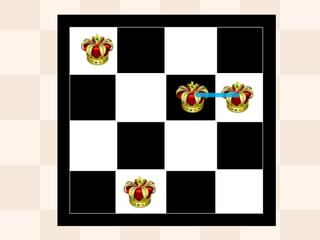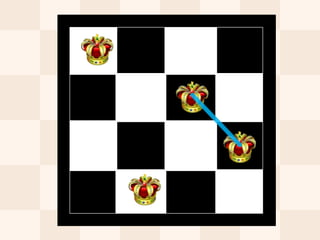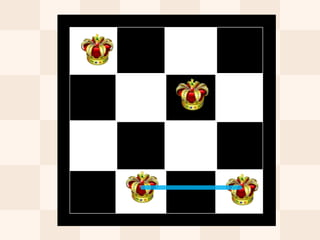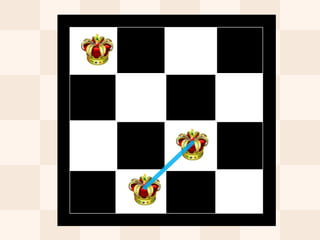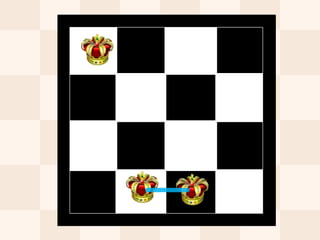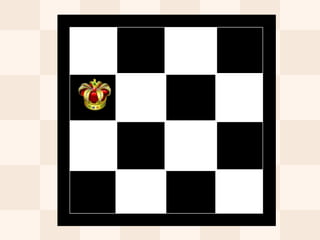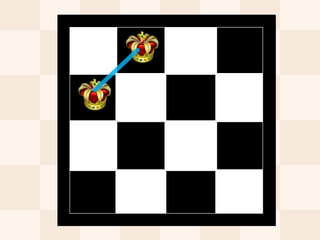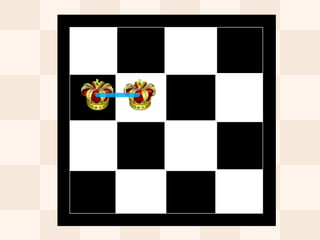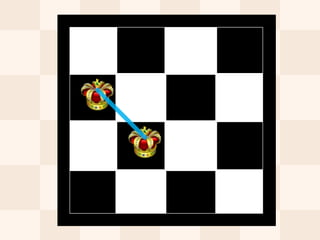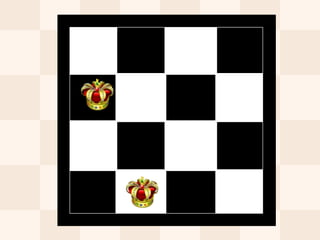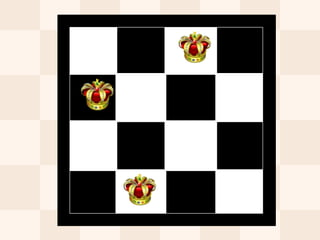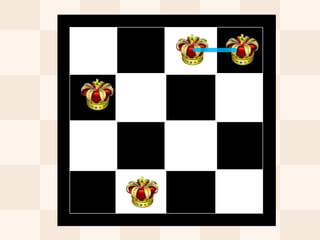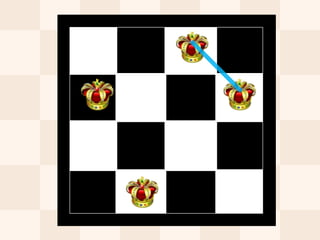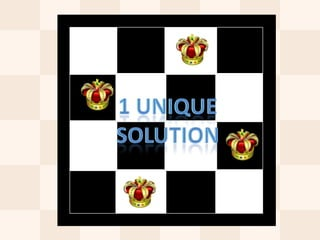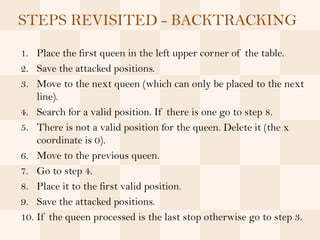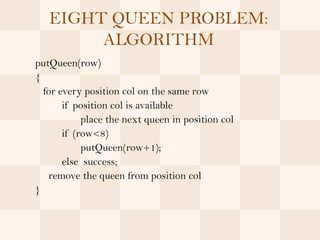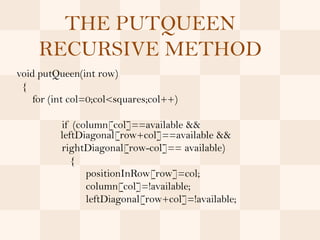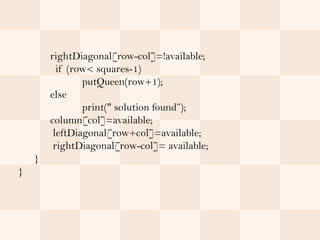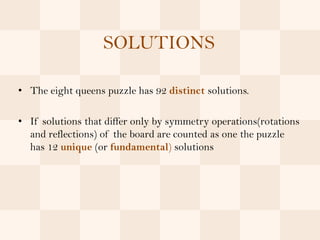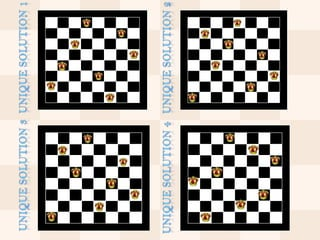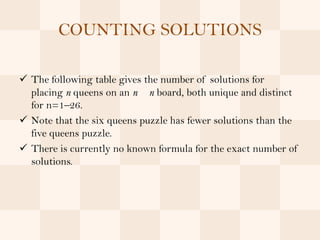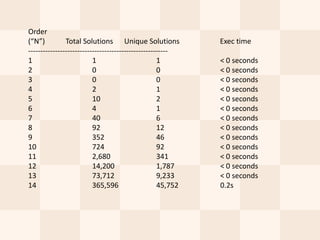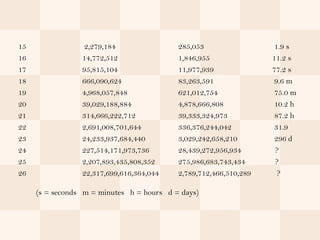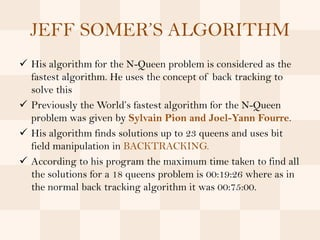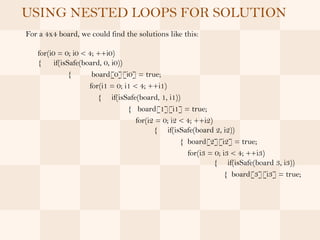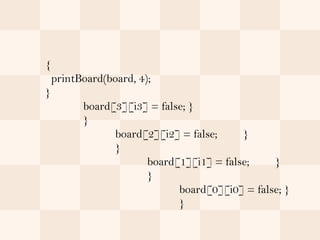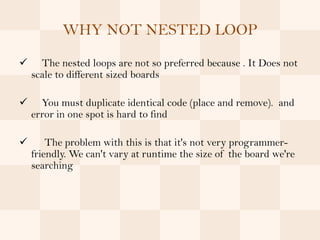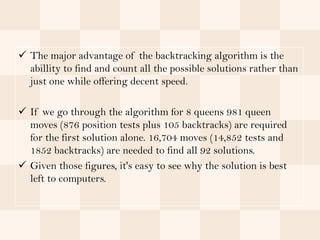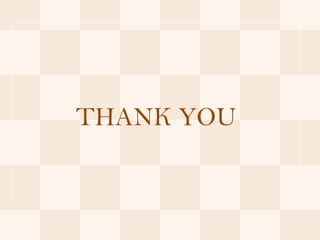1 of 51

## Recommended15 puzzle problem using branch and boundAbhishek SinghState Space Representation and SearchHitesh Mohapatra1.10. pumping lemma for regular setsSampath Kumar S15. Transactions in DBMSkoolkampusNp cooks theoremNarayana Galla

### 8 queens problem using back tracking

1. 8 QUEENS PROBLEM USING BACK TRACKING
2. BACK TRACKING  Backtracking is a general algorithm for finding all (or some) solutions to some computational problem, that incrementally builds candidates to the solutions, and abandons each partial candidate ‘c’ ("backtracks") as soon as it determines that ‘c’ cannot possibly be completed to a valid solution.  Backtracking is an important tool for solving constraint satisfaction problems, such as crosswords, verbal arithmetic, Sudoku, and many other puzzles.
3.  It is also the basis of the so-called logic programming languages such as Planner and Prolog.  The term "backtrack" was coined by American mathematician D. H. Lehmer in the 1950s.  The pioneer string-processing language SNOBOL (1962) may have been the first to provide a built-in general backtracking facility.
4.  The good example of the use of backtracking is the eight queens puzzle, that asks for all arrangements of eight queens on a standard chessboard so that no queen attacks any other.  In the common backtracking approach, the partial candidates are arrangements of k queens in the first k rows of the board, all in different rows and columns.  Any partial solution that contains two mutually attacking queens can be abandoned, since it cannot possibly be completed to a valid solution
5. WHAT IS 8 QUEEN PROBLEM?  The eight queens puzzle is the problem of placing eight chess queens on an 8 8 chessboard so that no two queens attack each other.  Thus, a solution requires that no two queens share the same row, column, or diagonal.  The eight queens puzzle is an example of the more general n-queens problem of placing n queens on an n n chessboard, where solutions exist for all natural numbers n with the exception of 1, 2 and 3.  The solution possibilities are discovered only up to 23 queen.
6. PROBLEM INVENTOR  The puzzle was originally proposed in 1848 by the chess player Max Bezzel, and over the years, many mathematicians, including Gauss, have worked on this puzzle and its generalized n-queens problem.
7. SOLUTION INVENTOR  The first solution for 8 queens were provided by Franz Nauck in 1850. Nauck also extended the puzzle to n-queens problem (on an n n board—a chessboard of arbitrary size).  In 1874, S. Günther proposed a method of finding solutions by using determinants, and J.W.L. Glaisher refined this approach.  Edsger Dijkstra used this problem in 1972 to illustrate the power of what he called structured programming.  He published a highly detailed description of the development of a depth-first backtracking algorithm.
8. Formulation : States: any arrangement of 0 to 8 queens on the board Initial state: 0 queens on the board Successor function: add a queen in any square Goal test: 8 queens on the board, none attacked
9. BACKTRACKING CONCEPT  Each recursive call attempts to place a queen in a specific column.  For a given call, the state of the board from previous placements is known (i.e. where are the other queens?)  Current step backtracking: If a placement within the column does not lead to a solution, the queen is removed and moved "down" the column  Previous step backtracking: When all rows in a column have been tried, the call terminates and backtracks to the previous call (in the previous column)
10. CONTINU.. Pruning: If a queen cannot be placed into column i, do not even try to place one onto column i+1 – rather, backtrack to column i-1 and move the queen that had been placed there. Using this approach we can reduce the number of potential solutions even more
11. BACKTRACKING DEMO FOR 4 QUEENS
12. STEPS REVISITED - BACKTRACKING 1. Place the first queen in the left upper corner of the table. 2. Save the attacked positions. 3. Move to the next queen (which can only be placed to the next line). 4. Search for a valid position. If there is one go to step 8. 5. There is not a valid position for the queen. Delete it (the x coordinate is 0). 6. Move to the previous queen. 7. Go to step 4. 8. Place it to the first valid position. 9. Save the attacked positions. 10. If the queen processed is the last stop otherwise go to step 3.
13. EIGHT QUEEN PROBLEM: ALGORITHM putQueen(row) { for every position col on the same row if position col is available place the next queen in position col if (row<8) putQueen(row+1); else success; remove the queen from position col }
14. THE PUTQUEEN RECURSIVE METHOD void putQueen(int row) { for (int col=0;col<squares;col++) if (column[col]==available && leftDiagonal[row+col]==available && rightDiagonal[row-col]== available) { positionInRow[row]=col; column[col]=!available; leftDiagonal[row+col]=!available;
15. rightDiagonal[row-col]=!available; if (row< squares-1) putQueen(row+1); else print(" solution found”); column[col]=available; leftDiagonal[row+col]=available; rightDiagonal[row-col]= available; } }
16. SOLUTIONS • The eight queens puzzle has 92 distinct solutions. • If solutions that differ only by symmetry operations(rotations and reflections) of the board are counted as one the puzzle has 12 unique (or fundamental) solutions
17. COUNTING SOLUTIONS  The following table gives the number of solutions for placing n queens on an n n board, both unique and distinct for n=1–26.  Note that the six queens puzzle has fewer solutions than the five queens puzzle.  There is currently no known formula for the exact number of solutions.
18. Order (“N”) Total Solutions Unique Solutions Exec time --------------------------------------------------------- 1 1 1 < 0 seconds 2 0 0 < 0 seconds 3 0 0 < 0 seconds 4 2 1 < 0 seconds 5 10 2 < 0 seconds 6 4 1 < 0 seconds 7 40 6 < 0 seconds 8 92 12 < 0 seconds 9 352 46 < 0 seconds 10 724 92 < 0 seconds 11 2,680 341 < 0 seconds 12 14,200 1,787 < 0 seconds 13 73,712 9,233 < 0 seconds 14 365,596 45,752 0.2s
19. 15 2,279,184 285,053 1.9 s 16 14,772,512 1,846,955 11.2 s 17 95,815,104 11,977,939 77.2 s 18 666,090,624 83,263,591 9.6 m 19 4,968,057,848 621,012,754 75.0 m 20 39,029,188,884 4,878,666,808 10.2 h 21 314,666,222,712 39,333,324,973 87.2 h 22 2,691,008,701,644 336,376,244,042 31.9 23 24,233,937,684,440 3,029,242,658,210 296 d 24 227,514,171,973,736 28,439,272,956,934 ? 25 2,207,893,435,808,352 275,986,683,743,434 ? 26 22,317,699,616,364,044 2,789,712,466,510,289 ? (s = seconds m = minutes h = hours d = days)
20. JEFF SOMER’S ALGORITHM  His algorithm for the N-Queen problem is considered as the fastest algorithm. He uses the concept of back tracking to solve this  Previously the World’s fastest algorithm for the N-Queen problem was given by Sylvain Pion and Joel-Yann Fourre.  His algorithm finds solutions up to 23 queens and uses bit field manipulation in BACKTRACKING.  According to his program the maximum time taken to find all the solutions for a 18 queens problem is 00:19:26 where as in the normal back tracking algorithm it was 00:75:00.
21. USING NESTED LOOPS FOR SOLUTION For a 4x4 board, we could find the solutions like this: for(i0 = 0; i0 < 4; ++i0) { if(isSafe(board, 0, i0)) { board[i0] = true; for(i1 = 0; i1 < 4; ++i1) { if(isSafe(board, 1, i1)) { board[i1] = true; for(i2 = 0; i2 < 4; ++i2) { if(isSafe(board 2, i2)) { board[i2] = true; for(i3 = 0; i3 < 4; ++i3) { if(isSafe(board 3, i3)) { board[i3] = true;
22. { printBoard(board, 4); } board[i3] = false; } } board[i2] = false; } } board[i1] = false; } } board[i0] = false; } }
23. WHY NOT NESTED LOOP  The nested loops are not so preferred because . It Does not scale to different sized boards  You must duplicate identical code (place and remove). and error in one spot is hard to find  The problem with this is that it's not very programmer- friendly. We can't vary at runtime the size of the board we're searching
24.  The major advantage of the backtracking algorithm is the abillity to find and count all the possible solutions rather than just one while offering decent speed.  If we go through the algorithm for 8 queens 981 queen moves (876 position tests plus 105 backtracks) are required for the first solution alone. 16,704 moves (14,852 tests and 1852 backtracks) are needed to find all 92 solutions.  Given those figures, it's easy to see why the solution is best left to computers.
25. THANK YOU